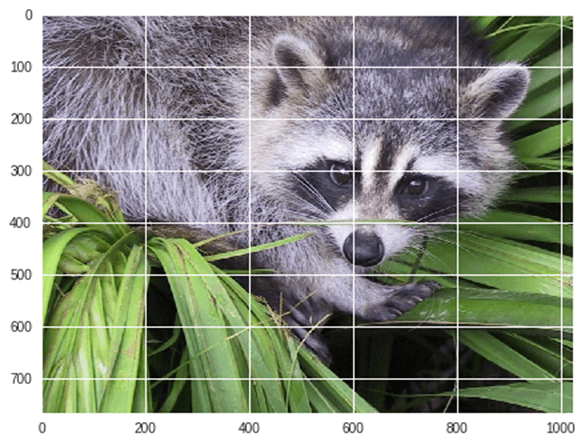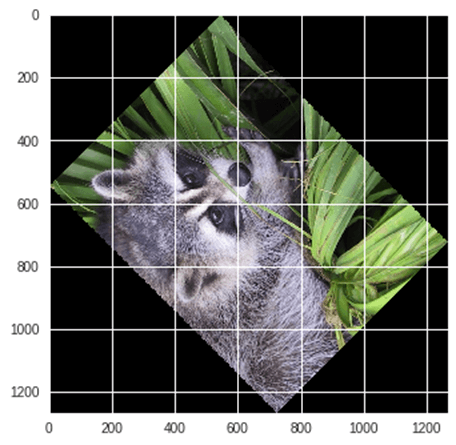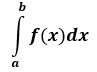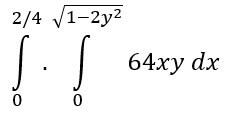Home » Blog » Image Processing with SciPy – scipy.ndimage

# Image Processing with SciPy – scipy.ndimage

## Image Processing with SciPy – scipy.ndimage

• scipy.ndimage is a submodule of SciPy which is mostly used for performing an image related operation
• ndimage means the “n” dimensional image.
• SciPy Image Processing provides Geometrics transformation (rotate, crop, flip), image filtering (sharp and de nosing), display image, image segmentation, classification and features extraction.
• MISC Package in SciPy contains prebuilt images which can be used to perform image manipulation task

Example: Let’s take a geometric transformation example of images

```from scipy import misc
from matplotlib import pyplot as plt
import numpy as np
#get face image of panda from misc package
panda = misc.face()
#plot or show image of face
plt.imshow( panda )
plt.show()```

Output:Now we Flip-down current image:

```#Flip Down using scipy misc.face image
flip_down = np.flipud(misc.face())
plt.imshow(flip_down)
plt.show()```

Output:Example: Rotation of Image using Scipy,

```from scipy import ndimage, misc
from matplotlib import pyplot as plt
panda = misc.face()
#rotatation function of scipy for image – image rotated 135 degree
panda_rotate = ndimage.rotate(panda, 135)
plt.imshow(panda_rotate)
plt.show()```

Output:### Integration with Scipy – Numerical Integration

• When we integrate any function where analytically integrate is not possible, we need to turn for numerical integration
• SciPy provides functionality to integrate function with numerical integration.
• scipy.integrate library has single integration, double, triple, multiple, Gaussian quadrate, Romberg, Trapezoidal and Simpson’s rules.

Example: Now take an example of Single IntegrationHere a is the upper limit and b is the lower limit

```from scipy import integrate
# take f(x) function as f
f = lambda x : x**2
#single integration with a = 0 & b = 1
integration = integrate.quad(f, 0 , 1)
print(integration)```

Output:

(0.33333333333333337, 3.700743415417189e-15)

Here function returns two values, in which the first value is integration and second value is estimated error in integral.

Example: Now take an example of double integration. We find the double integration of the following equation,```from scipy import integrate
import numpy as np
#import square root function from math lib
from math import sqrt
# set  fuction f(x)
f = lambda x, y : 64 *x*y
# lower limit of second integral
p = lambda x : 0
# upper limit of first integral
q = lambda y : sqrt(1 - 2*y**2)
# perform double integration
integration = integrate.dblquad(f , 0 , 2/4,  p, q)
print(integration)```

Output:

(3.0, 9.657432734515774e-14)

You have seen that above output as same previous one.